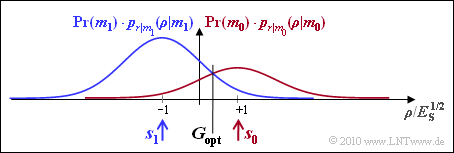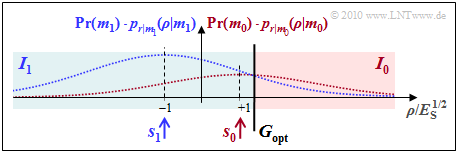# Exercise 4.07: Decision Boundaries once again

We consider a transmission system with

1. only one basis function  $(N = 1)$,
2. two signals  $(M = 2)$  with  $s_0 = \sqrt{E_s}$  and  $s_1 = -\sqrt{E_s}$ ,
3. an AWGN channel with variance  $\sigma_n^2 = N_0/2$.

Since this exercise deals with the general case   ${\rm Pr}(m_0) ≠ {\rm Pr}(m_1)$,  it is not sufficient to consider the conditional density functions   $p_{\it r\hspace{0.05cm}|\hspace{0.05cm}m_i}(\rho\hspace{0.05cm} |\hspace{0.05cm}m_i)$.  Rather,  these must still be multiplied by the symbol probabilities   ${\rm Pr}(m_i)$.  For   $i$,  the values  $0$  and  $1$  have to be used here.

If the decision boundary between the two regions  $I_0$  and  $I_1$  is at  $G = 0$,  i.e.,  in the middle between  $\boldsymbol{s}_0$  and  $\boldsymbol{s}_1$,  the error probability is independent of the occurrence probabilities  ${\rm Pr}(m_0)$  and  ${\rm Pr}(m_1)$:

$$p_{\rm S} = {\rm Pr}({ \cal E} ) = {\rm Q} \left ( \frac{d/2}{\sigma_n} \right ) \hspace{0.05cm}.$$
• Here  $d$  indicates the distance between the signal points  $s_0$  and  $s_1$  and  $d/2$  accordingly the respective distance of  $s_0$  and  $s_1$  from the decision boundary  $G = 0$.
• The rms value  (root of the variance)  of the AWGN noise is  $\sigma_n$.

If,  on the other hand,  the occurrence probabilities are different   ⇒  ${\rm Pr}(m_0) ≠ {\rm Pr}(m_1)$,  a smaller error probability can be obtained by shifting the decision boundary  $G$:

$$p_{\rm S} = {\rm Pr}(m_1) \cdot {\rm Q} \left ( \frac{d/2}{\sigma_n} \cdot (1 + \gamma) \right ) + {\rm Pr}(m_0) \cdot {\rm Q} \left ( \frac{d/2}{\sigma_n} \cdot (1 - \gamma) \right )\hspace{0.05cm},$$

where the auxiliary quantity  $\gamma$  is defined as follows:

$$\gamma = 2 \cdot \frac{ \sigma_n^2}{d^2} \cdot {\rm ln} \hspace{0.15cm} \frac{{\rm Pr}( m_1)}{{\rm Pr}( m_0)} \hspace{0.05cm},\hspace{0.2cm} G_{\rm opt} = \gamma \cdot \sqrt {E_{\rm S}}\hspace{0.05cm}.$$

Notes:

### Questions

1

What are the underlying symbol probabilities of the graph,  if the blue Gaussian curve is exactly twice as high as the red one?

 ${\rm Pr}(m_0)\ = \$ ${\rm Pr}(m_1)\ = \$

2

What is the error probability with noise variance  $\sigma_n^2 = E_{\rm S}/9$  and the  given threshold   $G = 0$?

 $G = 0 \text{:} \hspace{0.85cm} p_{\rm S} \ = \$ $\ \%$

3

What is the optimal threshold value for the given probabilities?

 $G_{\rm opt}\ = \$ $\ \cdot \sqrt{E_s}$

4

What is the error probability for  optimal threshold   $G = G_{\rm opt}$?

 $G = G_{\rm opt} \text{:} \hspace{0.2cm} p_{\rm S}\ = \$ $\ \%$

5

What error probabilities are obtained with the noise variance  $\sigma_n^2 = E_{\rm S}$?

 $G = 0 \text{:} \hspace{0.85cm} p_{\rm S}\ = \$ $\ \%$ $G = G_{\rm opt} \text{:} \hspace{0.2cm} p_{\rm S}\ = \$ $\ \%$

6

Which statements are true for the noise variance  $\sigma_n^2 = 4 \cdot E_{\rm S}$?

 With  $G = 0$,  the error probability is greater than  $30\%$. The optimal decision threshold is to the right of  $s_0$. With optimal threshold,  the error probability is about  $27\%$. The estimated value  $m_0$  is possible only with sufficiently large noise.

### Solution

#### Solution

(1)  The heights of the two drawn density functions are proportional to the occurrence probabilities ${\rm Pr}(m_0)$ and ${\rm Pr}(m_1)$. From

$${\rm Pr}(m_1) = 2 \cdot {\rm Pr}(m_0) \hspace{0.05cm},\hspace{0.2cm} {\rm Pr}(m_0) + {\rm Pr}(m_1) = 1$$
it follows directly  ${\rm Pr}(m_0) = 1/3 \ \underline {\approx 0.333}$  and  ${\rm Pr}(m_1) = 2/3 \ \underline {\approx 0.667}$.

(2)  With the decision boundary  $G = 0$,  independently of the occurrence probabilities:

$$p_{\rm S} = {\rm Q} \left ( \frac{d/2}{\sigma_n} \right ) \hspace{0.05cm}.$$
• With  $d = 2 \cdot \sqrt{E_{\rm S}}$  and  $\sigma_n = \sqrt{E_{\rm S}}/3$,  this gives:
$$p_{\rm S} = {\rm Q} (3) \hspace{0.1cm} \hspace{0.15cm}\underline {\approx 0.135 \%} \hspace{0.05cm}.$$

(3)  According to the specification,  for the  "normalized threshold":

$$\gamma = \frac{G_{\rm opt}}{E_{\rm S}^{1/2}} = 2 \cdot \frac{ \sigma_n^2}{d^2} \cdot {\rm ln} \hspace{0.15cm} \frac{{\rm Pr}( m_1)}{{\rm Pr}( m_0)} = \frac{ 2 \cdot E_{\rm S}/9}{4 \cdot E_{\rm S}} \cdot {\rm ln} \hspace{0.15cm} \frac{2/3}{1/3} \hspace{0.1cm}\hspace{0.15cm}\underline {\approx 0.04} \hspace{0.05cm}.$$
• Thus,  $G_{\rm opt} = \gamma \cdot \sqrt{E_{\rm S}} = 0.04 \cdot \sqrt{E_{\rm S}}$.
• Thus,  the optimal decision boundary is shifted to the right  $($towards the more improbable symbol  $s_0)$,  because  ${\rm Pr}(m_0) < {\rm Pr}(m_1)$.

(4)  With this optimal decision boundary,  the error probability is slightly smaller compared to subtask  (2):

$$p_{\rm S} \hspace{-0.1cm} \ = \ \hspace{-0.1cm} {2}/{3} \cdot {\rm Q} (3 \cdot 1.04) + {1}/{3} \cdot {\rm Q} (3 \cdot 0.96) = {2}/{3} \cdot 0.090 \cdot 10^{-2} + {1}/{3} \cdot 0.199 \cdot 10^{-2} \hspace{0.1cm}\hspace{0.15cm}\underline {\approx 0.126 \%} \hspace{0.05cm}.$$

(5)  With the decision boundary in the middle between the symbols  $(G = 0)$,  the result is analogous to subtask  (2)  with the noise variance now larger:Probability density functions with  $\sigma_n^2 = E_{\rm S}$
$$p_{\rm S} = {\rm Q} \left ( \frac{d/2}{\sigma_n} \right ) = {\rm Q} \left ( \frac{\sqrt{E_{\rm S}}}{\sqrt{E_{\rm S}}} \right )= {\rm Q} (1)\hspace{0.1cm} \hspace{0.15cm}\underline {\approx 15.9 \%} \hspace{0.05cm}.$$
• The parameter  $\gamma$  (normalized best possible displacement of the decision boundary)  is
$$\gamma = 2 \cdot \frac{ \sigma_n^2}{d^2} \cdot {\rm ln} \hspace{0.15cm} \frac{{\rm Pr}( m_1)}{{\rm Pr}( m_0)} = \frac{ 2 \cdot E_{\rm S}}{4 \cdot E_{\rm S}} \cdot {\rm ln} \hspace{0.15cm} \frac{2/3}{1/3} = \frac{{\rm ln} \hspace{0.15cm} 2}{2} \approx 0.35$$
$$\Rightarrow \hspace{0.3cm} G_{\rm opt} = 0.35 \cdot \sqrt{E_{\rm S}} \hspace{0.05cm}.$$
• The more frequent symbol is now less frequently falsified ⇒ the mean error probability becomes smaller:
$$p_{\rm S} = {2}/{3} \cdot {\rm Q} (1.35) + {1}/{3} \cdot {\rm Q} (0.65) = {2}/{3} \cdot 0.0885 +{1}/{3} \cdot 0.258 \hspace{0.1cm} \hspace{0.15cm}\underline {\approx 14.5 \%} \hspace{0.05cm}.$$
• The sketch makes it clear that the optimal decision boundary can also be determined graphically as intersection of the two  (weighted)  probability density functions:

(6)  All solutions  of this rather academic subtask  are correct:Probability density functions with  $\sigma_n^2 = 4 \cdot E_{\rm S}$
• With threshold  $G = 0$,  we get  $p_{\rm S} = {\rm Q}(0.5) \ \underline {\approx 0.309}$.
• The parameter  $\gamma = 1.4$  now has four times the value compared to subtask  (5),
so that the optimal decision boundary is now  $G_{\rm opt} = \underline {1.4 \cdot s_0}$.
• Thus,  the  (undisturbed)  KORREKTUR: (without noise) signal value  $s_0$  does not belong to the decision region  $I_0$,  but to  $I_1$,  characterized by  $\rho < G_{\rm opt}$.
• Only with a  (positive)  noise component:  $I_0 (\rho > G_{\rm opt})$  is possible at all.  For the error probability,  with  $G_{\rm opt} = 1.4 \cdot s_0$:
$$p_{\rm S} \hspace{-0.1cm} \ = \ \hspace{-0.1cm} {2}/{3} \cdot {\rm Q} (0.5 \cdot (1 + 1.4)) + {1}/{3} \cdot {\rm Q} (0.5 \cdot (1 - 1.4)) = \hspace{0.15cm}\underline {\approx 27\%} \hspace{0.05cm}.$$
• The accompanying graph illustrates the statements made here.